### YIELD Function – Calc Bond Yield – Excel, VBA, & G Sheets

This tutorial demonstrates how to use the Excel YIELD Function in Excel to calculate the bond yield.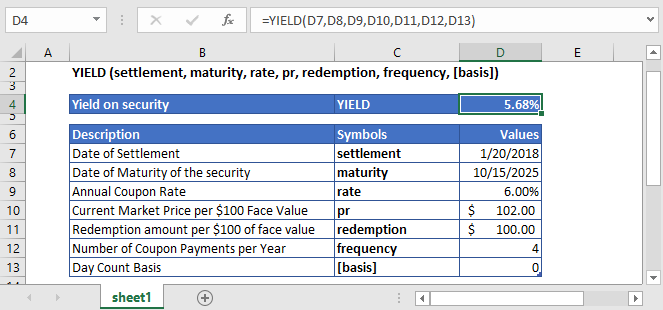## YIELD Function Overview

The YIELD Function Calculates the bond yield.

To use the YIELD Excel Worksheet Function, select a cell and type: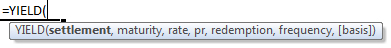(Notice how the formula inputs appear)

### YIELD Function Syntax and Inputs:

`=YIELD(settlement, maturity, rate, pr, redemption, frequency, [basis])`

settlement – It’s the settlement date of the security or the date at which the security is purchased. It’s the date that comes after the issuing date of the security.

maturity – It’s the date at which the bond or security expires, and the principal amount is paid back to bond or security holder.

rate – It’s the annual interest rate of the bond or security at which the coupon payments are processed.

pr – It’s the face value of the security or the bond. If this argument is omitted, then the YIELD function will assume the par value to be \$1,000.

redemption – The bond’s or security’s redemption value per \$100 face value that is reimbursed to bond or security holder on the redemption date.

frequency – It refers to the number of periodic coupon payments per year. The value of frequency for annual, semi-annual, and quarterly payments are 1, 2, and 4, respectively.

basis – OPTIONAL. It specifies the type of day counting to be used by the security or bond. Possible values can be:

 Basis Day Count 0 US (NASD) 30/360 1 Actual/actual 2 Acutal/360 3 Acutal/365 4 European 30/360

If the basis argument is omitted, it assumes its default value i.e. US (NASD) 30/360.

AutoMacro - VBA Code Generator

## What Is YIELD?

The Yield on security is the amount of cash earned on security in the form of interest or dividends. It is expressed as a percentage based on the face value of the security.

YIELD of a bond is calculated using the following formula if there is no more than one coupon period until the redemption.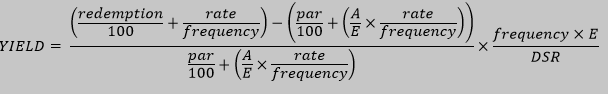Where:

DSR = number of days from the settlement date to the redemption date

E = number of days in coupon period in which the settlement date falls

A = number of days from the beginning of the coupon period to the settlement date.

If more than one coupon is remaining until the redemption, then YIELD is calculated through iterations. YIELD function uses the PRICE formula to solve for the yield using the Newton method (also known as the Newton-Raphson method).

## What Is Excel YIELD Function?

The Excel YIELD function returns the yield on a security that pays periodic interest. It returns the value as a percentage.

### Calculate the Yield of a security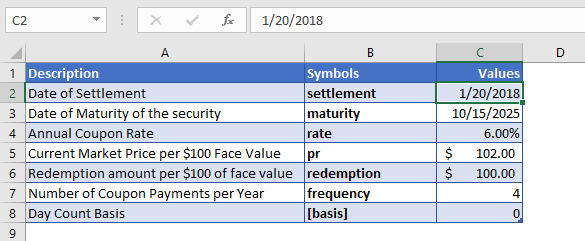In this example, we calculate the yield on a security that was purchased on January 20, 2018, with an annual interest rate of 6%. Other details of the security are mentioned in the above table.

The formula used to calculate the Yield is:

`=YIELD(C4,C5,C6,C7,C8,C9,C10)`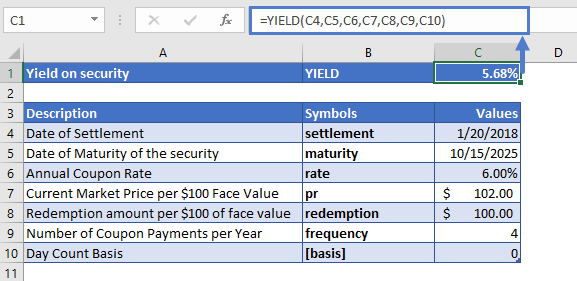The YIELD function returns the yield of security:

YIELD = 5.68%

Here the price of the security argument (pr) and redemption argument (redemption) is entered as the value per \$100 regardless of the actual face value of the security. Also as recommended the values of the settlement and maturity date arguments are entered as a reference to the cells containing dates.

### Calculate Yield of a 10 Year Bond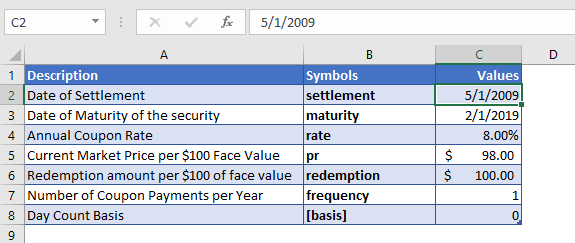Now let’s calculate the yield of a 10-year bond, which was issued on February 1, 2009, and was purchased by the investor three months later. Other details of the bond are mentioned in the above table.

The formula used to calculate the Yield is:

`=YIELD(C4,C5,C6,C7,C8,C9,C10)`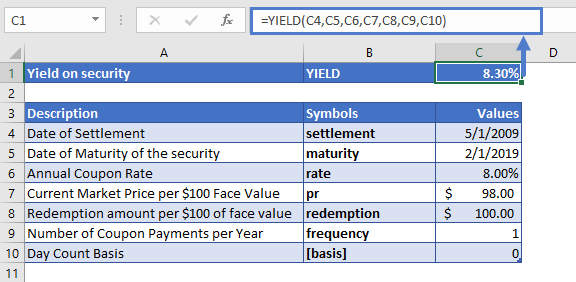The YIELD function calculates the yield of the 10-year bond:

YIELD = 8.3%

As recommended the values of the settlement and maturity date arguments are entered as a reference to the cells containing dates.

## YIELD in Google Sheets

All of the above examples work exactly the same in Google Sheets as in Excel.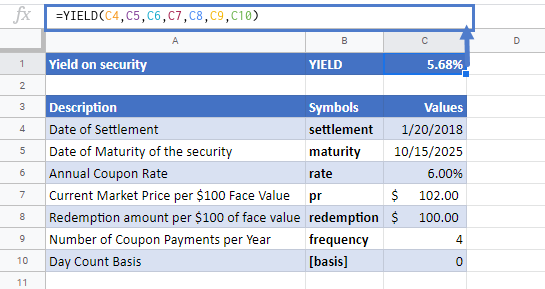## Additional Notes

Use the YIELD Function to calculate the yield of a bond or similar security.

#NUM! Error occurs if the settlement date is greater or equal than the maturity date; or the values of the rate, redemption, frequency, or [basis] arguments are not valid numbers (i.e. rate < 0; or pr ≤ 0; or redemption ≤ 0; or frequency is any value other than 1, 2 or 4; or [basis] value is other than 0, 1, 2, 3, or 4)

#VALUE! Error occurs if the dates of the settlement or the maturity arguments are not valid Excel dates.

It is recommended that the settlement and maturity dates should be entered in the YIELD function as references to cells containing the dates or dates returned from formulas.

The result of the YIELD Function may appear as a decimal. Change the cell Number Formatting to Percentage to display the function result as a percentage:Return to the List of all Functions in Excel

## YIELD Examples in VBA

You can also use the YIELD function in VBA. Type:
`application.worksheetfunction.yield(settlement,maturity,rate,pr,redemption,FREQUENCY,basis)`
For the function arguments (rate, etc.), you can either enter them directly into the function or define variables to use instead.

## Excel Practice Worksheet

Practice Excel functions and formulas with our 100% free practice worksheets!

• Automatically Graded Exercises
• Learn Excel, Inside Excel!